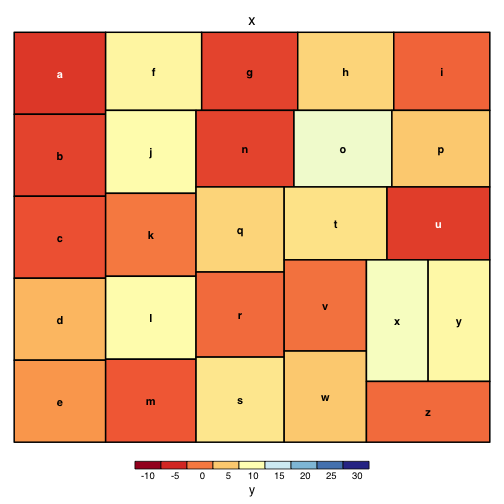# treemap: mapping to color palettes

This small tutorial is about the mapping of values to color palettes, for treemap types “value” and “manual”.

There are two arguments that determine the mapping to color palettes: `mapping` and `palette`. The arguent `range` does not influence this mapping, as it only defines what range of color values is shown in the legend.

The only difference between “value” and “manual” is the default value for `mapping`. The “value” treemap considers `palette` to be a diverging color palette (say ColorBrewer’s `"RdYlBu"`), and maps it in such a way that 0 corresponds to the middle color (typically white or yellow), `-max(abs(values))` to the left-end color, and `max(abs(values))`, to the right-end color. The “manual” treemap simply maps `min(values)` to the left-end color, `max(values)` to the right-end color, and `mean(range(values))` to the middle color.

The following fictive data is created:

``````dat <- data.frame(letters=letters[1:26], x=1, y=runif(26)*16-4)
``````

### “value” type treemaps

#### `mapping=c(-max(abs(values)), 0, max(abs(values)))`

``````treemap(dat, index="letters", vSize="x", vColor="y", type="value", palette="RdYlBu")
``````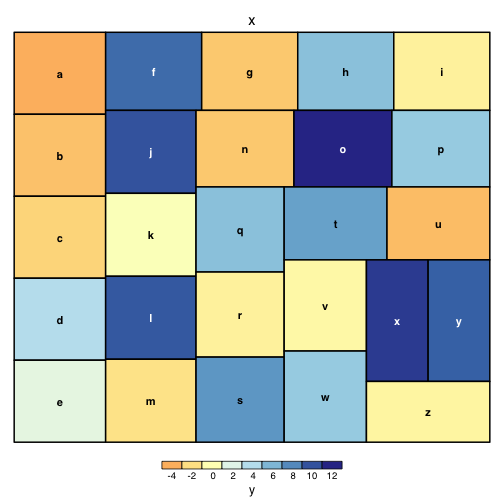You see that the center color (Yl) will automatically be assigned to 0, negative values to Rd-Yl and positive to Yl-Bu. Since `min(dat\$y)` is `-3.86` and `max(dat\$y)` is `11.77`, the applied mapping is `c(-11.7, 0, 11.7)`. The reason why you see only -4 to 12 in the legend (and not -12 to 12), is because the `range` argument is by default c(min(values), max(values)) with some pretty rounding. This can be changed of course:

``````treemap(dat, index="letters", vSize="x", vColor="y", type="value", palette="RdYlBu", range=c(-12,12), n = 9)
``````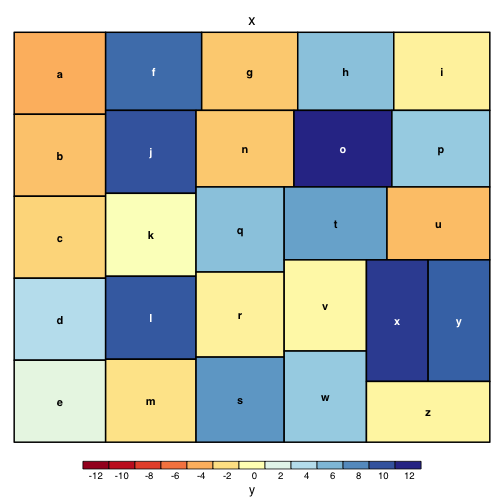### “manual” type treemaps

#### `mapping=c(min(values), mean(range(values)), max(values))`

``````treemap(dat, index="letters", vSize="x", vColor="y", type="manual", palette="RdYlBu")
``````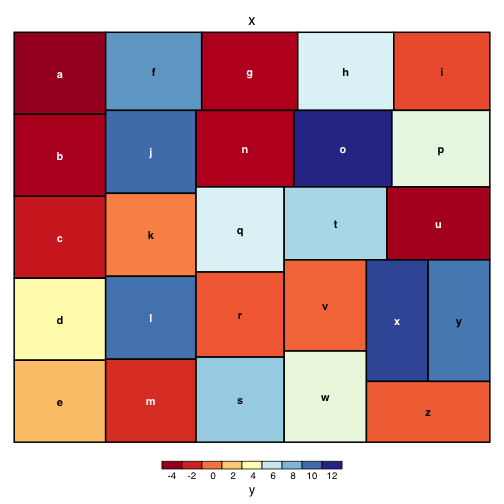The “manual” type does not interpret the values as the “value” type does. Instead, the value range is mapped linearly to the color palette. In this case, the middle color yellow is assigned to (-3.86 + 11.77) / 2, which is 3.955.

### custom mapping

When the mapping argument is specified, the “value” and “manual” treemap types are identical. Say we assign -10 to red, 10 to yellow and 30 to blue:

``````treemap(dat, index="letters", vSize="x", vColor="y", type="value", palette="RdYlBu",
mapping=c(-10, 10, 30))
``````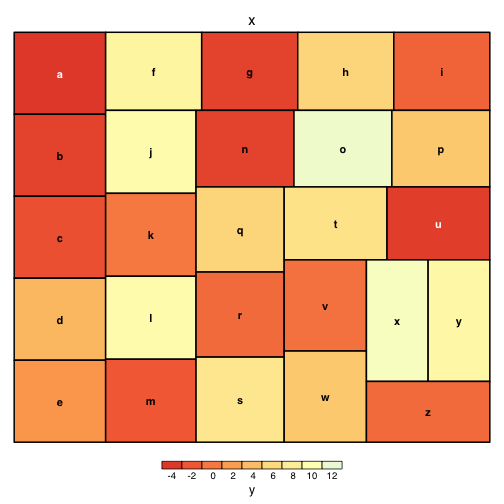Almost all values, and hence the legend, are mapped to the lefthand-side of the palette. To see the full mapping in the legend, use the “range” argument:

``````treemap(dat, index="letters", vSize="x", vColor="y", type="value", palette="RdYlBu",
mapping=c(-10, 10, 30), range=c(-10, 30))
``````